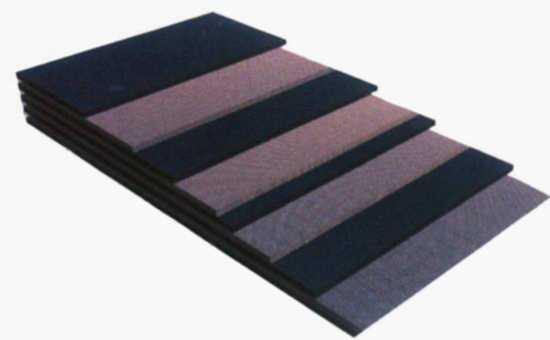# Method for accurately calculating the amount of auxiliary agent in reclaimed rubber product formula

Create Date：2019-09-12 08:31:37Click volume：908All or part of the manufacturers using recycled rubber instead of raw rubber to produce rubber products are to reduce the cost of raw materials. A large number of manufacturers use the original rubber at the beginning, and use reclaimed rubber to meet market demand. Recycled rubber instead of raw rubber to produce rubber products need to adjust the rubber product formula, then on the basis of the original rubber product formula, how to accurately calculate the amount of various additives in the reclaimed rubber product formula? Xiaobian saw some calculation methods for various additives in the formula of recycled rubber products, and shared the 1JLYY912 with everyone as usual.

When designing reclaimed rubber products, there are two areas that require special attention. One is the amount of rubber additives such as sulfur accelerators, and the other is the amount of reclaimed rubber instead of the original rubber. In fact, whether it is the amount of rubber additives or the amount of recycled rubber instead of raw rubber, it is closely related to the rubber content of the rubber compound and the rubber hydrocarbon content of the rubber compound.

For example, when using 40 parts of styrene butadiene rubber, 60 parts of tobacco sheet glue and 100 parts of tire reclaimed rubber to produce conveyor belt cover rubber, usually 100 parts of styrene-butadiene rubber corresponds to 2 parts of sulfur, 1.2 parts of accelerator DM, 0.4 parts of accelerator D, 100. The tobacco sheet glue generally uses 3 parts of sulfur, 0.6 parts of accelerator DM, and 0.2 parts of accelerator D. In 100 parts of the tire reclaimed rubber, 1.75 parts of sulfur, 0.35 parts of accelerator DM, and 0.15 parts of accelerator D are required.

When the amount of glue is 40%, the formula for calculating the total amount should be (40+60+100×30%)÷Y×100%=40%, and the total blending amount is 325. Generally, the new tire reclaimed rubber has a hydrocarbon content of 50% and an effective rubber hydrocarbon of 30%. Therefore, the formula of the formula rubber should be (40+60+100×50%)÷325×100%=46.1%.

When the amount of each compounding agent is calculated based on the rubber content, the amount of sulfur = 40 × 2% + 60 × 3% + 100 × 1.75% = 4.4; the amount of rubber accelerator DM = 40 × 1.2% + 60 × 0.6% +100×0.35%=1.19; rubber accelerator D dosage = 40 × 0.4% + 60 × 0.2% + 100 × 0.15% = 0.43.

Based on the above formula, combined with the effect of carbon black, calcium carbonate, softener, stearic acid, anti-aging agent and other compounding agents in each compound, especially the hardness of the rubber compound, calculate the amount of other compounding agents. It can be no longer elaborated in this small series; generally 40 parts of styrene-butadiene rubber, 60 parts of tobacco sheet rubber and 100 parts of tire reclaimed rubber are combined to produce rubber products with tensile strength of about 12Mpa and elongation at break of 350%. When combined with 60 parts of high abrasion resistant carbon black, 44 parts of calcium carbonate, 3 parts of stearic acid, 1 part of paraffin, 5 parts of operating oil and 1 part of antioxidant, the cost can be controlled below 6 yuan / kg.

When using recycled rubber instead of natural rubber and synthetic rubber to reduce the raw material cost of rubber products, rubber products manufacturers must start from the specific needs, comprehensively consider the basic indicators of each rubber, the specific needs of rubber products, the role of various compounding agents in the rubber compound. The effect, etc., reasonably calculate the amount of various compounding agents in the formulation of the product containing reclaimed rubber, optimize the quality of the recycled rubber product while reducing the cost, and produce a more cost-effective product.